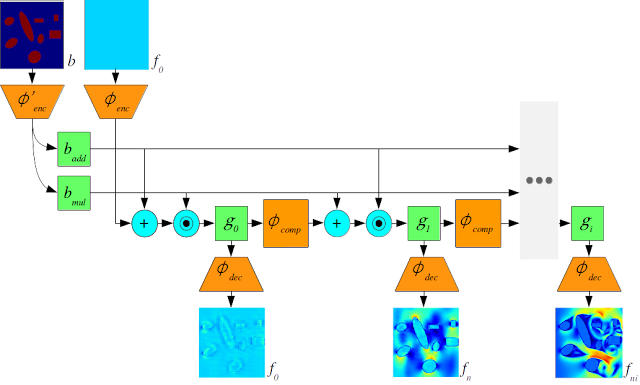## Monday, November 13, 2017

### Deep Learning to Accelerate Computational Fluid DynamicsLat-Net: Compressing Lattice Boltzmann Flow Simulations using Deep Neural Networks
I posted about a surprising application of deep learning to accelerate topology optimization. The thing I like about that approach is it's a strategy that could be applied to accelerate many different solvers that we use to simulate all sorts of continuum mechanics based on partial differential equations (i.e. computational fluid dynamics, structural mechanics, electrodynamics, etc.). With a bit of help from Google I found a neat paper and project on github doing exactly that for a Lattice-Boltzmann fluid solver.

Here's the abstract,
Computational Fluid Dynamics (CFD) is a hugely important subject with applications in almost every engineering field, however, fluid simulations are extremely computationally and memory demanding. Towards this end, we present Lat-Net, a method for compressing both the computation time and memory usage of Lattice Boltzmann flow simulations using deep neural networks. Lat-Net employs convolutional autoencoders and residual connections in a fully differentiable scheme to compress the state size of a simulation and learn the dynamics on this compressed form. The result is a computationally and memory efficient neural network that can be iterated and queried to reproduce a fluid simulation. We show that once Lat-Net is trained, it can generalize to large grid sizes and complex geometries while maintaining accuracy. We also show that Lat-Net is a general method for compressing other Lattice Boltzmann based simulations such as Electromagnetism.

This is pretty cool. Other similar efforts,
have shown orders of magnitude reduction in run-time for the price of an approximation error and the upfront cost of training the network. There's a short discussion on Reddit about this as well.